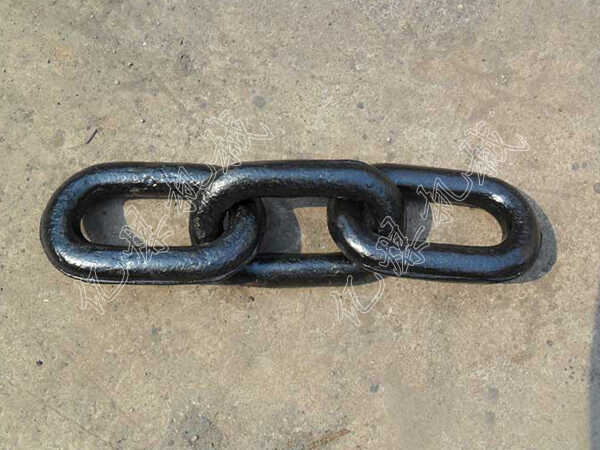# 你知道三环链的拉力验算吗

=900*0.75*1.1

=742.5kg

=1700*0.75*1.1

=1402.5kg

1、φ16.2极限荷重 280KN      280000/9.8=28571.43Kg

原煤车: 28571.43Kg/4237.2Kg =6.74 ＜10

2、φ24.6极限荷重406KN      406000/9.8=41428.57kg

原煤车: 41428.57kg /4237.2Kg =9.78 ＜  10

3、φ26.9极限荷重468KN      468000/9.8=47755.10kg

原煤车: 47755.10kg //4237.2Kg =11.27 ＞ 10

4、φ29.4极限荷重430KN      430000/9.8=43877.55kg

原煤车: 43877.55kg/4237.2Kg =10.36 ＞ 10

5、φ31.6极限荷重440KN     440000/9.8=44897.96kg

原煤车: 44897.96kg /4237.2Kg =10.60 ＞ 10